GeeksforGeeks App
Open AppBrowser
Continue

## Related Articles

• NCERT Solutions for Class 8 Maths

# Class 8 NCERT Solutions – Chapter 5 Data Handling – Exercise 5.2

### Problem 1. A survey was made to find the type of music that a certain group of young people liked in a city. The adjoining pie chart shows the findings of this survey. From this pie chart answer the following:

• If 20 people liked classical music, how many young people were surveyed?
• Which type of music is liked by the maximum number of people?
• If a cassette company were to make 1000 CD’s, how many of each type would they make?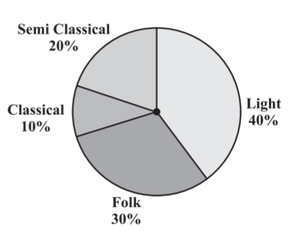Solution:

(i) Given, 20 people liked classical music. From the pie chart, we can see that classical music is liked by 10% of young people in the city. Let the total number of people in the city = x

Given, 10% of x = 20

(10/100) * x = 20

x = (20 * 100) / 10 = 200

So, 200 young people were surveyed.

(ii) Since light music occupies a maximum percentage (40%) in the pie chart, it is liked by the maximum number of people.

(iii) If a cassette company were to make 1000 CD’s, they would make

Classical music CDs = 10% of 1000

=  (10 / 100) * 1000

= 100 CDs

Semi Classical music CDs = 20% of 1000

= (20 / 100) * 1000

= 200 CDs

Folk music CDs = 30% of 1000

= (30 / 100) * 1000

= 300 CDs

Light music CDs = 40% of 1000

= (40 / 100) * 1000

= 400 CDs

### Problem 2. A group of 360 people was asked to vote for their favorite season from the three seasons rainy, winter, and summer.

• Which season got the most votes?
• Find the central angle of each sector.
• Draw a pie chart to show this information.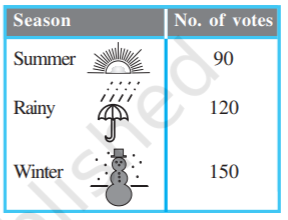Solution:

(i) Winter season got the most votes (150).

(ii) Find the proportion and percentage of each sector to calculate the central angle of each sector.

Total votes = 90 + 120 + 150 = 360

Proportion of summer season = 90 / 360 = 1 / 4, Percentage = (1 / 4) * 100 = 25%

Proportion of rainy season = 120 / 360 = 1 / 3, Percentage = (1 / 3) * 100 = 33.334%

Proportion of winter season = 150 / 360 = 5 / 12, Percentage = (5 / 12) * 100 = 41.667%

Now, the central angle of each sector is,

Summer season = (25 / 100) * 360° = 90°

Rainy season = (33.334 / 100) * 360° = 120°

Winter season = (41.667 / 100) * 360° = 150°

(iii) Pie Chart: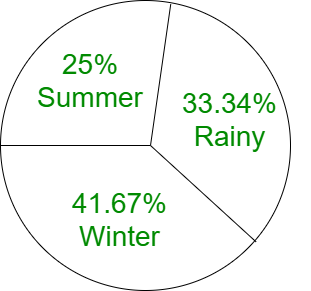### Problem 3. Draw a pie chart showing the following information. The table shows the colors preferred by a group of people.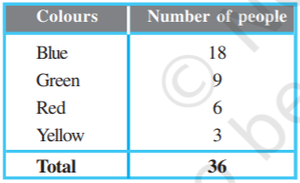Solution:

Find the proportion and percentage of each sector to calculate the central angle of each sector.

Total number of people = 18 + 9 + 6 + 3 = 36

Proportion of Blue = 18 / 36 = 1 / 2, Percentage = (1 / 2) * 100 = 50%

Proportion of Green = 9 / 36 = 1 / 4, Percentage = (1 / 4) * 100 = 25%

Proportion of Red = 6 / 36 = 1 / 6, Percentage = (1 / 6) * 100 = 16.667%

Proportion of Yellow = 3 / 36 = 1 / 12, Percentage = (1 / 12) * 100 = 8.334%

Now, the central angle of each sector is,

Blue = (50 / 100) * 360° = 180°

Green= (41.667 / 100) * 360° = 90°

Red = (16.667 / 100) * 360° = 60°

Yellow = (8.334 / 100) * 360° = 30°

Pie Chart: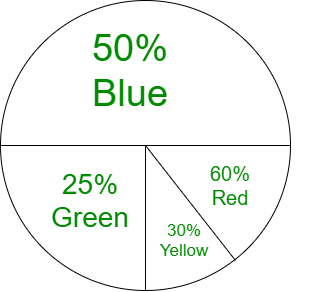### Problem 4. The adjoining pie chart gives the marks scored in an examination by a student in Hindi, English, Mathematics, Social Science, and Science. If the total marks obtained by the students were 540, answer the following questions.

• In which subject did the student score 105 marks?
• How many more marks were obtained by the student in Mathematics than in Hindi?
• Examine whether the sum of the marks obtained in Social Science and Mathematics is more than that in Science and Hindi.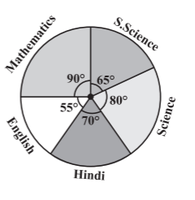Solution:

(i)  Given, total marks obtained by the students were 540, So,

For 540 marks, the central angle = 360°

For 1 mark, the central angle = (360 / 540)°

For 105 marks, the central angle = ((360 / 540) * 105)°

= 70°

From the given pie chart, 70° corresponds to Hindi language. Therefore, the student score 105 marks in   Hindi.

(ii) In mathematics, marks scored = (90 / 360) * 540

= 135 marks

In hindi, marks scored = (70 / 360) * 540

= 105 marks

Therefore, the student scored (135 – 105) = 30 marks more in Mathematics than in Hindi.

(iii) In mathematics, marks scored = 135 marks

In hindi, marks scored = 105 marks

In social science, marks scored  = (65 / 360) * 540

= 97.5 marks

In science, marks scored  = (80 / 360) * 540

= 120 marks

Marks in social science + mathematics = 97.5 + 135 = 232.5

Marks in science + hindi = 120 + 105 = 225

232.5 > 225

Yes, the sum of the marks obtained in Social Science and Mathematics is more than that in Science and     Hindi.

Alternative way:

Central angle of social science + mathematics =  65° + 90° = 155°

Central angle of science + hindi = 80° + 70° = 150°

155° > 150°

Yes, the sum of the marks obtained in Social Science and Mathematics is more than that in Science and Hindi.

### Problem 5. The number of students in a hostel, speaking different languages is given below. Display the data in a pie chart.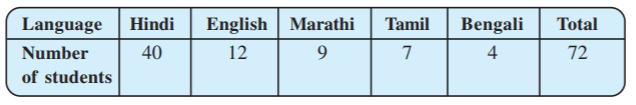Solution:

Find the proportion and percentage of each sector to calculate the central angle of each sector.

Total number of students = 72

Proportion of Hindi = 40 / 72 = 5 / 9, Percentage = (5 / 9) * 100 = 55.556%

Proportion of English = 12 / 72 = 1 / 6, Percentage = (1 / 6) * 100 = 16.667%

Proportion of Marathi = 9 / 72 = 1 / 8, Percentage = (1 / 8) * 100 = 12.5%

Proportion of Tamil = 7 / 72 = 7 / 72, Percentage = (7 / 72) * 100 = 9.723%

Proportion of Bengali = 4 / 72 = 1 / 18, Percentage = (1 / 18) * 100 = 5.556%

Now, the central angle of each sector is,

Hindi = (55.556 / 100) * 360° = 200°

English = (16.667 / 100) * 360° = 60°

Marathi = (12.5 / 100) * 360° = 45°

Tamil = (9.723 / 100) * 360° = 35°

Bengali = (5.556 / 100) * 360° = 20°

Pie Chart: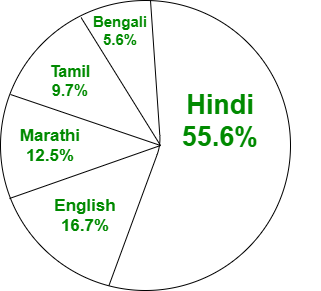My Personal Notes arrow_drop_up
Related Tutorials